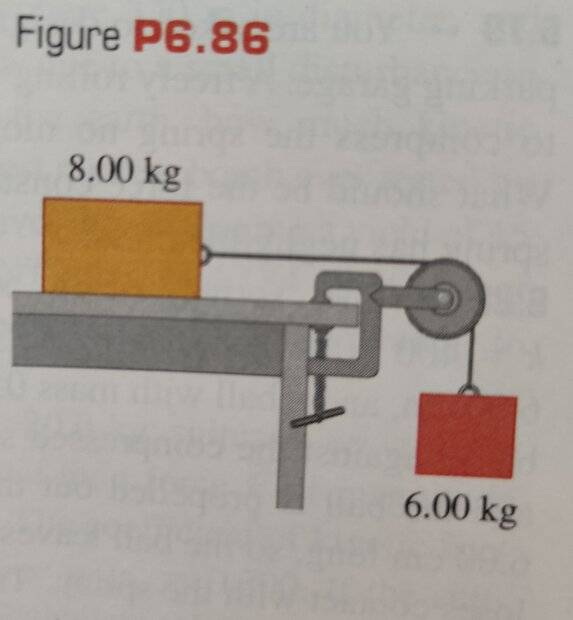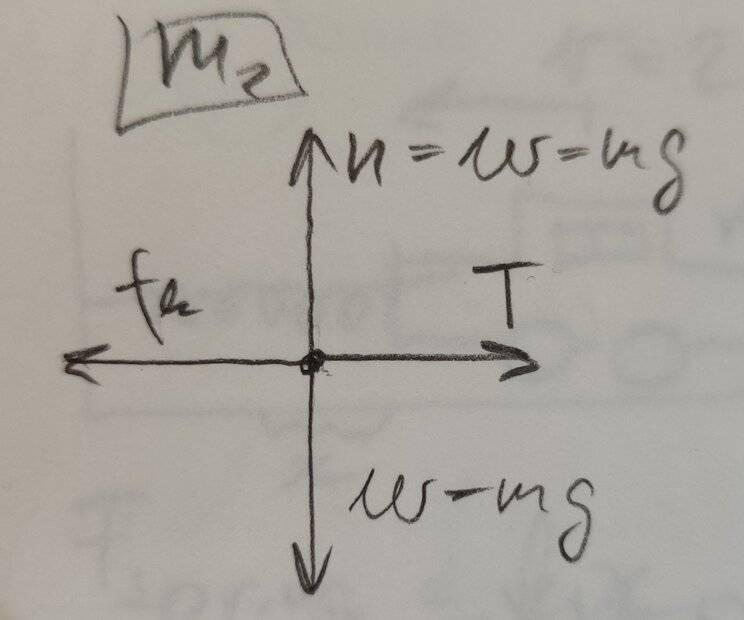# Work-Energy Theorem and Friction

• Argonaut
In summary, the problem involves two blocks, one moving vertically and one moving horizontally, with given masses, initial velocity, and displacement. The target variable is the coefficient of kinetic friction. Using the work-energy theorem and the balance of forces, the given values can be used to calculate the coefficient of kinetic friction, which is found to be 0.786. However, the book gives a slightly different value of 0.786, leading to further investigation and adjustments in the calculation to arrive at the correct value.f

#### Argonaut

Homework Statement
[Young & Freedman - University Physics 13th ed, exercise 6.87]
Consider the system shown in Fig. P6.86. The rope and pulley have negligible mass, and the pulley is frictionless. Initially the 6.00-kg block is moving downward and the 8.00-kg block is moving to the right, both with a speed of 0.900 m/s. The blocks come to rest after moving 2.00 m. Use the work–energy theorem to calculate the coefficient of kinetic friction between the 8.00-kg block and the tabletop.
Relevant Equations
Work-energy theorem
Kinetic friction formulaMy final answer is different from the official one in the back of the book, and I can't figure out what I did wrong. This is my attempt:

Let block 1 be the vertically moving block and let block 2 be the horizontally moving one.

Also, let ##m_1 = 6.00 ~\rm{kg}##, ##m_2 = 8.00 ~\rm{kg}##, ##v_0 = 0.900 ~\rm{m/s}##, and ##d = 2.00 ~\rm{m}##. The target variable is ##\mu_k##.

According to the work-energy theorem, the total work done on block 2 is ##W_{tot}= \Delta K = K_f - K_i = 0 -K_i = -\frac{1}{2}m_2v_0^2##.Alternatively, since the forces acting on block 2 are constant, we also have ##W_{tot} = \Sigma F_x d = (T - f_k)d = (m_1g - \mu_km_2g)d## (if the direction of displacement is the positive direction).

So then we have

$$-\frac{1}{2}m_2v_0^2 = (m_1g - \mu_km_2g)d$$

Rearranging to express ##\mu_k##, we obtain

$$\mu_k = \frac{m_1}{m_2} + \frac{v_0^2}{2dg} = \frac{6.00 ~\rm{kg}}{8.00 ~\rm{kg}} + \frac{(0.900 ~\rm{m/s})^2}{2(2.00 ~\rm{m})(9.80 ~\rm{m/s^2})} = 0.771$$

However, the book gives ##0.786##. Where did I mess up?

Alternatively, since the forces acting on block 2 are constant, we also have ##W_{tot} = \Sigma F_x d = (T - f_k)d = (m_1g - \mu_km_2g)d## (if the direction of displacement is the positive direction).

However, the book gives ##0.786##. Where did I mess up?
Consider the balance of forces on m1 and its resulting acceleration to find T.

But the easier way is to consider the whole of system energy.

••nasu and Argonaut
Consider the balance of forces on m1 and its resulting acceleration to find T.

But the easier way is to consider the whole of system energy.
Right, so since there is acceleration, Newton's First Law does not apply, and ##T \neq m_1g ##, in fact ## T > m_1g ##, is that correct?

In which case, I'm probably better off considering the whole system instead of block 2.

, in fact T>m1g, is that correct?
Does m1 rise?

Does m1 rise?
It does not, but its descent is 'slowing', so there is negative acceleration (supposing the positive direction is the direction of displacement). I thought that would mean that the force acting in the negative direction is greater than the one acting in the positive direction.

It does not, but its descent is 'slowing', so there is negative acceleration (supposing the positive direction is the direction of displacement). I thought that would mean that the force acting in the negative direction is greater than the one acting in the positive direction.
Sorry… skipping between threads, I forgot that it is initially descending.

•Argonaut
Sorry… skipping between threads, I forgot that it is initially descending.
No worries, and thanks for the help. Looking forward to my lunch break to have another go at this exerciseThe problem says
Initially the 6.00-kg block is moving downward and the 8.00-kg block is moving to the right, both with a speed of 0.900 m/s.
You say $$-\frac{1}{2}m_2v_0^2 = (m_1g - \mu_km_2g)d.$$
What should ##\Delta K## be?

•Argonaut and erobz
The problem says

You say $$-\frac{1}{2}m_2v_0^2 = (m_1g - \mu_km_2g)d.$$
What should ##\Delta K## be?
Thanks for the tip. I think I've got it now - at least I've managed to reproduce the correct value.

So on the one hand ##W_{tot} = \Delta K = K_f - K_i = 0 - \frac{1}{2}(m_1+m_2)v_0^2##.

On the other hand, treating the blocks, the rope and the pulley as a system, there are two forces doing work on the system. So we have ## W_{tot} = \Sigma F d = (w_1 - f_k)d = (m_1g - \mu_km_2g)d ##.

So then equating the two we obtain

$$(m_1g - \mu_km_2g)d = - \frac{1}{2}(m_1+m_2)v_0^2$$

$$\mu_k = \frac{m_1}{m_2} + \frac{(m_1+m_2)v_0^2}{2dm_2g} = \frac{6.00 ~\rm{kg}}{8.00 ~\rm{kg}} + \frac{(6.00 ~\rm{kg} + 8.00 ~\rm{kg})(0.900 ~\rm{m/s})^2}{2(2.00 ~\rm{m})(8.00 ~\rm{kg})(9.80 ~\rm{m/s^2})} = 0.786$$

That is correct.

•Argonaut